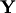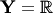# Probabilistic Regression

In probabilistic regression problems, the label spaceis the set of real numbers,, and Forecaster is required to output a probability measure on. The probability measure may be represented as its distribution function. An example of a forecasting strategy for such problems is conformal predictive systems.